# An object of mass 'm' is being moved with a constant velocity under the action of an applied force of 2N along a frictionless surface with following surface profile.Question:

An object of mass 'm' is being moved with a constant velocity under the action of an applied force of 2N along a frictionless surface with following surface profile.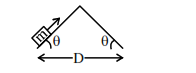The correct applied force vs distance graph will be:

1.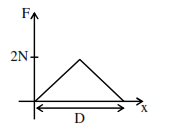2.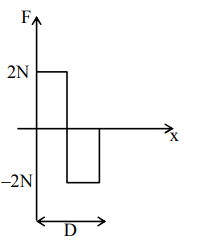3.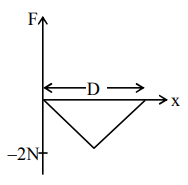4.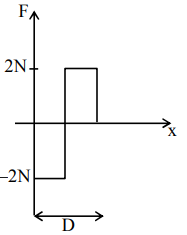Correct Option: , 2

Solution:

During upward motion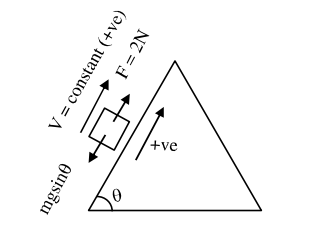F = 2N = (+ve) constant During downward motion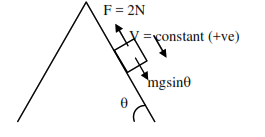$\Rightarrow \mathrm{F}=2 \mathrm{~N}=(-\mathrm{ve})$ constant

$\Rightarrow$ Best possible answer is option (2)